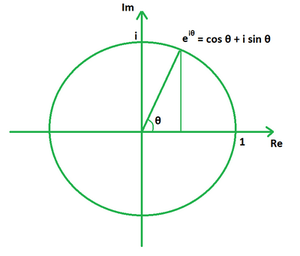GeeksforGeeks App
Open AppBrowser
Continue

## Related Articles

Euler’s formula holds a prominent place in the field of mathematics. It aids in establishing the essential link between trigonometric functions and complex exponential functions. It is a crucial formula used for solving complicated exponential functions. It is also known as Euler’s identity. It has a lot of applications in complex analysis and is used to find the number of vertices and faces of a polyhedral.

Formula for Complex Analysis

The complex analysis formula provides us with a way to express the imaginary power of the exponential functions in form of trigonometric ratios. Its formula can be related to a complex plane, where a unit complex function eix traces a unit circle, such that x is a real number measured in radians.eix = cos x + i sin x

where,

x is a real number,

e is the logarithm’s base,

sin x and cos x are the trigonometric functions,

i is the imaginary part.

The expression cos x + i sin x is also known as polar form of a complex number.

Derivation

Consider the expansion series of the exponential function ex.

ex = 1 + x + x2/2! + x3/3! + x4/4! + …….. + ∞

Substituting x as ix we get,

eix = 1 + ix + (ix)2/2! + (ix)3/3! + (ix)4/4! + …….. + ∞

Using the property i2 = -1 we get,

= 1 + ix – x2/2! – ix3/3! + x4/4! + …….. + ∞

= (1 – x2/2! + x4/4! – …….. + ∞) + i (x – x3/3! + x5/5! – …….. + ∞)

Using series expansion property of sine and cosine functions we get,

= cos x + i sin x

This derives the Euler’s formula for complex analysis.

Formula for Polyhedral

The polyhedral formula of Euler states that the number of faces, vertices, and edges of every polyhedron that does not self-intersect are connected in a certain way. Its formula says that the number of vertices and faces of a polyhedral combined is two greater than its number of edges.

F + V – E = 2

where,

F is the number of faces,

V the number of vertices,

E the number of edges.

Derivation

Euler’s formula can be proven for five platonic solids: cube, tetrahedron, octahedron, dodecahedron and the icosahedron.

### Sample Problems

Problem 1. Express eiπ/2 in the general form using Euler’s formula.

Solution:

We have,

x = π/2

Using the formula we get,

eix = cos x + i sin x

= cos π/2 + i sin π/2

= 0 + i (1)

= 0 + i

Problem 2. Express e6i in the general form using Euler’s formula.

Solution:

We have,

x = 6

Using the formula we get,

eix = cos x + i sin x

= cos 6 + i sin 6

= 0.96 + i (-0.279)

= 0.96 – 0.279i

Problem 3. Express e10i in the general form using Euler’s formula.

Solution:

We have,

x = 10

Using the formula we get,

eix = cos x + i sin x

= cos 10 + i sin 10

= -0.83 + i (-0.544)

= -0.83 – 0.544i

Problem 4. Express eiπ/3 in the general form using Euler’s formula.

Solution:

We have,

x = π/3

Using the formula we get,

eix = cos x + i sin x

= cos π/3 + i sin π/3

= 0.5 + i (0.86)

= 0.5 + 0.86i

Problem 5. Verify Euler’s formula for a triangular prism.

Solution:

We have a triangular prism. It is known that,

Number of faces (F) = 5

Number of vertices (V) = 6

Number of edges (E) = 9

Using the formula we have,

F + V − E = 5 + 6 − 9

= 11 – 9

= 2

As the value of F + V − E is 2, the Euler’s formula is verified.

Problem 6. Verify Euler’s formula for a square pyramid.

Solution:

We have a square pyramid. It is known that,

Number of faces (F) = 5

Number of vertices (V) = 5

Number of edges (E) = 8

Using the formula we have,

F + V − E = 5 + 5 − 8

= 10 – 8

= 2

As the value of F + V − E is 2, the Euler’s formula is verified.

Problem 7. Find the number of vertices of a polyhedral if the number of faces is 20 and the edges is 30.

Solution:

We have,

F = 20

E = 30

Using the formula we get,

F + V − E = 2

=> V = E + 2 – F

= 30 + 2 – 20

= 32 – 20

= 12

My Personal Notes arrow_drop_up
Related Tutorials